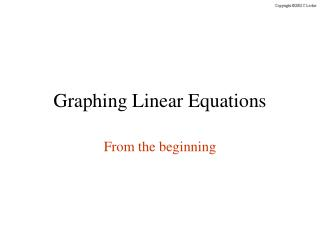DownloadDownload PresentationGraphing Linear Equations

# Graphing Linear Equations

Télécharger la présentation## Graphing Linear Equations

- - - - - - - - - - - - - - - - - - - - - - - - - - - E N D - - - - - - - - - - - - - - - - - - - - - - - - - - -
##### Presentation Transcript

1. Graphing Linear Equations From the beginning

2. Click the mouse button to advance the slide when you see this icon. • All the slides in this presentation are timed. • You do not need to click the mouse or press any keys on the keyboard for the presentation on each slide to continue. • However, in order to make sure the presentation does not go too quickly, you will need to click the mouse or press a key on the keyboard to advance to the next slide. • You will know when the slide is finished when you see a small icon in the bottom left corner of the slide.

3. y x Linear Not Linear What is a Linear Equation? A linear equation is an equation whose graph is a LINE.

4. y x What is a Linear Equation? The equations we will be graphing have two variables, x and y. For example, A solution to the equation is any ordered pair (x , y) that makes the equation true. The ordered pair (3 , 2) is a solution since, If we were to plot all these ordered pairs on a graph, we would be graphing a line.

5. y x The x - values are picked by YOU! Graphing a Linear Equation How do we graph linear equations? Let’s try this one: y = 3x – 2 Make a Table of values –8 y = 3(–2) – 2 = –8 Complete the table by inputting the x - values and calculating the corresponding y - values. –5 y = 3(–1) – 2 = –5 –2 y = 3(0) – 2 = –2 1 y = 3(1) – 2 = 1 4 y = 3(2) – 2 = 4

6. y x Graphing a Linear Equation How about another one! Let’s try x – 2y = 5. First Step: Write y as a function of x x – 2y = 5 –2y = 5 – x

7. y x Take a moment and complete the chart… Click the screen when finished Graphing a Linear Equation How about another one! Let’s try x – 2y = 5. Second Step: Make a Table of Values –3 –2

8. y x Graphing Horizontal & Vertical Lines When you are asked to graph a line, and there is only ONE variable in the equation, the line will either be vertical or horizontal. For example … Graph x = 3 y = –2 Since there are no y – values in this equation, x is always 3 and y can be any other real number. Graph y = –2 Since there are no x – values in this equation, y is always – 2 and x can be any other real number. x = 3